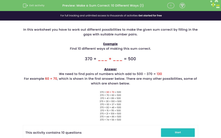# Make an Addition Sum Correct

In this worksheet, students will find ten number pairs to make a sum correct, initially using subtraction to find the amount missing.Key stage:  KS 2

Curriculum topic:   Number: Addition and Subtraction

Curriculum subtopic:   Solve Add/Subtract Problems to 1000

Difficulty level:#### Worksheet Overview

In this activity, you have to work out different possibilities to make the given sum correct by filling in the gaps with suitable number pairs.

Example

Find ten different ways of making this sum correct.

370 + ___ + ___ = 500

We need to find pairs of numbers which add to 500 - 370 = 130

For example 60 + 70, which is shown in the first answer below. There are many other possibilities, some of which are shown below.

370 + 60 + 70 = 500

370 + 70 + 60 = 500

370 + 41 + 89 = 500

370 + 30 + 100 = 500

370 + 93 + 37 = 500

370 + 82 + 48 = 500

370 + 15 + 115 = 500

370 + 21 + 109 = 500

370 + 44 + 86 = 500

370 + 74 + 56 = 500

Let's try some questions now.

### What is EdPlace?

We're your National Curriculum aligned online education content provider helping each child succeed in English, maths and science from year 1 to GCSE. With an EdPlace account you’ll be able to track and measure progress, helping each child achieve their best. We build confidence and attainment by personalising each child’s learning at a level that suits them.

Get started